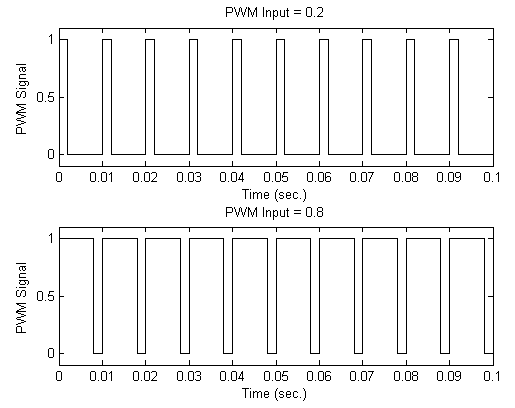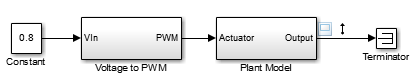## Configure Models with Pulse Width Modulation Signals

Many industrial applications use pulse width modulation (PWM) signals because such signals are robust in the presence of noise. When using Simulink® Control Design™ software, subsystems that contain PWM signals do not linearize well due to discontinuities in the signal.

The following figure shows two PWM signals. The top plot shows a PWM signal with a 20% duty cycle, which represents a 0.2 V DC signal. The signal is 1 V for 20% of each cycle and 0 V for the remaining 80% of the cycle. The average signal value is 0.2 V. The bottom plot shows a PWM signal with an 80% duty cycle, which represents a 0.8 V DC signal.For an example of such a PWM system, open the `scdpwm` model. In this model, a constant signal is converted to a PWM signal using the Voltage to PWM subsystem.

`open_system('scdpwm')`In this model, a constant signal is converted to a PWM signal using the Voltage to PWM subsystem.

`open_system('scdpwm/Voltage to PWM')`When linearizing a model that contains PWM signals there are two effects that produce poor linearization results:

• The signal level at the operating point is one of the discrete values within the PWM signal, not the DC signal value. For example, in the `scdpwm` model, the signal level is either 0 or 1, not 0.8. This change in operating point affects the linearized model.

• The creation of the PWM signal within the `Voltage to PWM` subsystem uses the Compare To Zero block. Such comparator blocks do not linearize well due to their discontinuities.

To linearize a model that contains PWM signals, you must replace the linearization of the blocks or subsystems that produce the PWM signals. To do so, use one of the following methods:

### Functions